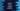# Python program to check if a number is duck number or not## Duck number program in Python:

This post will show you how to check if a number is a duck number or not in Python. A number is called a duck number if the number contains at least one zero and it doesn’t start with a zero. We can also say that a positive number that has at least one zero is called a duck number.

For example, 12034 is a duck number because it has one zero. Similarly, 123004 is also a duck number. But 01234 is not a duck number as it starts with a zero. 012304 is a duck number because it has one zero even if we don’t consider the leading zero.

To check if a number is a duck number or not, we must check if the number contains a non-leading zero digit. We can write a program in different ways in Python.

### Method 1: By iterating through the digits of the number:

We can iterate through the digits of the number one by one to check if the number is a duck number. The loop will ignore the leading zeroes. Below is the complete algorithm:

• Take the number as input from the user.
• Iterate over the digits of the number one by one.
• If it finds any zero in the number, it will be a duck number. Else, it is not a duck number.

Below is the complete program:

``````def is_duck(n):
i = 0

while i < len(n) and n[i] == '0':
i += 1

while i < len(n):
if n[i] == '0':
return True
i += 1

return False

if __name__ == '__main__':
test_number_array = ["0", "0000", "0123", "1230", "123", "1203", "120034", "012340"]

for number in test_number_array:
if is_duck(number):
print(f'{number} is a duck number')
else:
print(f'{number} is not a duck number')``````

Here,

• The is_duck method is used to check if a number is a duck number or not. It takes one number as its parameter and returns one Boolean value.
• The first while loop is used to iterate over the leading zeroes.
• The second while loop iterates over other digits of the number and checks if there is any zero in the number. If it find any zero, it returns True. Else, if it doesn’t find any zero, it returns False.
• The test_number_array is an array of numbers. The for loop iterates over these numbers and checks if each number is a duck number or not.

It will print the below output:

``````0 is not a duck number
0000 is not a duck number
0123 is not a duck number
1230 is a duck number
123 is not a duck number
1203 is a duck number
120034 is a duck number
012340 is a duck number``````

### Method 2: By using lstrip:

The lstrip method is used to remove any leading character from a string in python. This method is defined as below:

``str.lstrip(characters)``

Here, characters are the characters we want to remove from the start of a string. This is an optional value and if we don’t pass this value, it will remove the spaces. This method returns the new string.

By using the lstrip method, we can remove all leading zeroes from a string and we can check if there is any zero in the remaining part of the string or not.

Below is the complete program:

``````def is_duck(n):
n_lstrip = n.lstrip("0")

if "0" in n_lstrip:
return True
return False

if __name__ == '__main__':
test_number_array = ["0", "0000", "0123", "1230", "123", "1203", "120034", "012340"]

for number in test_number_array:
if is_duck(number):
print(f'{number} is a duck number')
else:
print(f'{number} is not a duck number')``````

In this example, we changed the is_duck method. It uses lstrip to remove all leading zeroes. This value is stored in the variable n_lstrip. The if block checks if there is any zero in n_lstrip or not. If yes, it returns True. Else, it returns False.

It will print the below output:

``````0 is not a duck number
0000 is not a duck number
0123 is not a duck number
1230 is a duck number
123 is not a duck number
1203 is a duck number
120034 is a duck number
012340 is a duck number``````